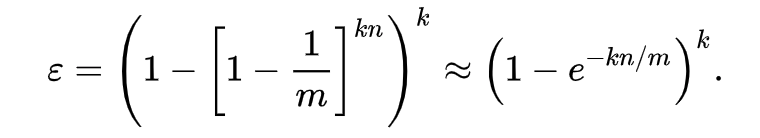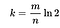# Bloom Filter: A Short Introduction

Bloom filter is a probabilistic data structure designed to tell you if a member is in a set in a highly memory and time efficient manner. In that sense, it is similar to a set, but it does it with an adjustable false positive rate. In bloom filter, false positives are possible, but false negatives are not. In other words, when testing if a member is in a set, it either says “possibly in a set” or “definitely not in a a set”. When it says yes, it might be wrong with an often small enough chance, but when it says no, that means no.

A brilliant example is provided in this blog post.

Bloom filter is especially fit for large set, where it is hard to fit the whole set into main memory as it is, and when it is okay to allow certain rate of false positives.

One typical example is account name selection while creating your gmail account. You might wonder how does google know so fast that if an email account is taken in a blink? Think about it, Gmail has around 1.5 billions users, it is impossible to search through all of them. Nor it is ideal to store all those existing email addresses in main memory.

Instead, we can use a bit array with some help from a few hash functions to encode existing email addresses. When a user types a desired new address, we just need to check if the hash outputs (indices in the bit array) are all 1’s. If they answer is yes, there is high probability this address is already taken, otherwise, this address is definitely not taken.

The false positive rate is approximated by:From wikipedia

where m is number of bits in the bit array, n is the number of elements in the existing set, k is the number of hash functions.

To minimize the false positive rate given fixed m and n, we can choose the number of hash functions following:From wikipedia

# Notes:

1. For the calculation of False Positive rate: see wikipedia: https://en.wikipedia.org/wiki/Bloom_filter#Probability_of_false_positives
2. For an interactive example: https://llimllib.github.io/bloomfilter-tutorial
3. For another easy read on Medium: https://medium.com/techspace-usict/bloom-filter-a-probabilistic-search-approach-in-data-structures-cb3dd48c6632
4. A python C extension: http://axiak.github.io/pybloomfiltermmap/ref.html

Machine Learning & Software Engineer in Amsterdam, Holland